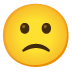How would one datashade the points / bivariate scatters in a hv.GridMatrix? I have tried mapping over the whole gridmatrix and calling holoviews.operation.datashader only on objects of type hv.Points, but the resulting object appears to be a dynamicMap and bokeh doesn’t know how to plot that.

Could you provide an example of what you’ve tried? What you’ve tried definitely should work.

It is good to hear that datashading should work.
But I cannot make it run with “Linked Selection Tools”, like Lasso Select.

The following is a self-contained example example which shows how it works well without datashading and then the problems arising with datashading:

``````import xarray as xr
import numpy as np
import holoviews as hv
from holoviews import opts
from holoviews.operation import gridmatrix, decimate
hv.extension("bokeh")

data = xr.merge([xr.DataArray(np.random.randn(100), dims=("x"), coords={"x": range(100)}, name="da_{}".format(i)) for i in range(4)])

gm_input = hv.Dataset(data.to_dataframe())
gm = gridmatrix(gm_input, diagonal_type=hv.Scatter).opts(opts.Scatter(frame_width=150, frame_height=150, size=2, tools=['box_select','lasso_select'], active_tools=['lasso_select'], fill_alpha=0.2, selection_color="red"))
gm
``````

Awesome X-Y plot! Now let’s datashade:
`gm.map(datashade, hv.Scatter)`

Unfortunately, with the previous set diagonal_type=to hv.Scatter, neither mapping with datashade nor decimate works. Former produces a `ValueError: repeated axis in transpose` the latter a `DataError: Dimensions may not reference duplicated DataFrame columns (found duplicate 'da_0' columns). ....`.
So plotting the same dimension onto each other doesn’t work so easy. Let’s simply change
diagonal_type=hv.Histogram and voila, datashading is fine!

But now we lost the awesome linked selection capabilities like the Lasso Selection ToolClear, the opts.Scatter is not applied to datashaded figures, but how would an alternative look like?

Giving an example how to make this selection linking work within a datashaded gridmatrix (maybe also taking the linking of the histograms into account) would be an immense educational example on how to manipulate/navigate through the holoviews world as it covers quite a lot I guess.